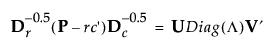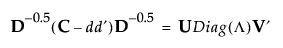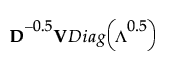Publication date: 11/29/2021

## Details Report

When a simple Correspondence Analysis is performed, the report lists the singular values from the following singular value decomposition:where:

P is the matrix of counts divided by the total frequency

r and c are the row and column sums of P

the D matrices are diagonal matrices of the values of r and c

Λ is the column vector of singular values

When Multiple Correspondence Analysis is performed, the singular value decomposition extends to the following equation:where:

C is the Burt table

d is a column vector of the column sums of C (d is also the row sums, since C is symmetric)

D is a diagonal matrix of the values of d

In the Details report, the inertia is the column vector Λ. The singular value is the square root of the inertia vector. The column coordinates are calculated as follows: